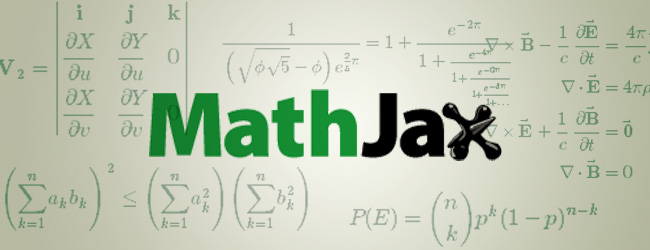Mathjax logo and interface screenshots © Mathjax

This is the second in a multi-part article about displaying mathematical notation within web pages.  In this part I discuss a modern solution to tackling the problem of displaying mathematics on the web.  If you are not already familiar with the issues surrounding this topic, you might find it useful to start with part 1.

## Part 2 – Beautiful mathematics in all browsers

About this time last year (January 2010), I received an email from a colleague in the School of Mathematics looking for a better way to present mathematics via a web browser.  For many users across the University, relying on very simple mathematical typesetting was just about enough, but he was a “power user” – Mathematics was his business!  I had a number of criteria in mind that I felt were important:

• Minimal setup overhead for the user
• Broad cross browser support with no plugin required
• Natural and fast method of input – text based (for faster input) with graphical editor support as a fall back
• The encoded notation should be as human readable as possible
• The encoded notation sit with the output document so the two never get separated
• Reliable and robust output format(s) with consideration of accessibility issues
• Compatibility with University supported VLEs

You could summarise the above as “I want something that ‘just works everywhere’ which is easy to work with”.  This is quite a tall order…

I focused my efforts on searching for a good Javascript display engine for mathematics and quickly settled upon four different implementations – ASCIIMathML, LaTeXMathML, jsMath, and MathJax.  It was apparent very early on that MathJax offered the best hope at meeting most of my criteria.  The other three implementations were much older and didn’t perform well in my tests and besides, MathJax was a complete updated reworking of jsMath by the same author.

### Introducing MathJax…

If you’ve read this far, well done, time to get down to business and talk about MathJax.  MathJax was built from ground up from 6 years prior experience by Davide Cervone the author of jsMath (a similar mathematics display tool) and was formally launched in 2010.  It has sponsors and supporters including the American Mathematical Society, Design Science (the main commercial sponsor) and The American Physical Society, but is an open source project and completely free to use.  Notable features also include:

• MathJax works in all modern browsers (including many mobile device browsers) without users needing to install any special browser extension or plugin.
• It provides high-quality typography as it uses modern CSS and web fonts, instead of equation images or Flash, so equations scale with surrounding text at all zoom levels.  Where older less compatible browsers are used, MathJax degrades gracefully and uses images to display mathematical characters and symbols.
• MathJax is compatible with screen readers used by people with vision disabilities, but also has a Zoom feature which helps all readers to see small details such as scripts, primes and hats.
• One can also copy and paste the web page mathematics into many mathematical software applications such as Mathematica and paste directly into Microsoft Word 2007 documents.

On the face of it, MathJax feels like it is the right technology at the right time.  With real competition between browser vendors, web browsers are becoming much faster and more standards compliant (even the lamentable Internet Explorer) and this makes innovations such as MathJax possible.

### MathJax in action…

The following equations are all displayed using MathJax, they are not images. Right-clicking on the mathematical reveals further display options (discussed further later).

#### The Cauchy-Schwarz Inequality

$$\left( \sum_{k=1}^n a_k b_k \right)^2 \leq \left( \sum_{k=1}^n a_k^2 \right) \left( \sum_{k=1}^n b_k^2 \right)$$

#### A Cross Product Formula

$$\mathbf{V}_1 \times \mathbf{V}_2 = \begin{vmatrix} \mathbf{i} & \mathbf{j} & \mathbf{k} \\ \frac{\partial X}{\partial u} & \frac{\partial Y}{\partial u} & 0 \\ \frac{\partial X}{\partial v} & \frac{\partial Y}{\partial v} & 0 \end{vmatrix}$$

#### The probability of getting k heads when flipping n coins is

$$P(E) = {n \choose k} p^k (1-p)^{ n-k}$$

#### An Identity of Ramanujan

$$\frac{1}{\Bigl(\sqrt{\phi \sqrt{5}}-\phi\Bigr) e^{\frac25 \pi}} = 1+\frac{e^{-2\pi}} {1+\frac{e^{-4\pi}} {1+\frac{e^{-6\pi}} {1+\frac{e^{-8\pi}} {1+\ldots} } } }$$

#### Maxwell’s Equations

\begin{aligned} \nabla \times \vec{\mathbf{B}} -\, \frac1c\, \frac{\partial\vec{\mathbf{E}}}{\partial t} & = \frac{4\pi}{c}\vec{\mathbf{j}} \\ \nabla \cdot \vec{\mathbf{E}} & = 4 \pi \rho \\ \nabla \times \vec{\mathbf{E}}\, +\, \frac1c\, \frac{\partial\vec{\mathbf{B}}}{\partial t} & = \vec{\mathbf{0}} \\ \nabla \cdot \vec{\mathbf{B}} & = 0 \end{aligned}

### How do I use MathJax?

MathJax is easy to use, but you may find it useful to read the documentation on the MathJax site or seek support from the EPS eLearning team to get started more quickly. Some very basic prior knowledge about how web page documents are laid out helps, but this can be easily picked up along the way.  In this article, only the very basics of how to use MathJax are covered, which for most people is sufficient.

#### Linking MathJax into a web page

MathJax uses the web technology JavaScript to reliably display mathematical notation across all modern browsers.  You can include MathJax in your web page by putting:

<script type="text/javascript" src="path-to-MathJax/MathJax.js"></script>

…in your document’s <head> block. Here, path-to-MathJax should be replaced by the URL for the main MathJax directory.  The MathJax directory might reside at a central location to which you can link to, or you may choose to download it and include it in the same directory as your web files.  In addition to linking to MathJax, there are a number of optional configuration settings which are beyond the scope of this article.

#### Putting mathematics in a web page

To put mathematics in your web page, you can use either LaTeX notation, or MathML notation (or both); the configuration file tells MathJax which you want to use.

Many mathematical software packages claim to support MathML by allow expressions to be exported as MathML or for MathML expressions to be pasted into the software.  Exported MathML can be pasted directly into the web document and all that is needed for MathJax to recognise it is to mark your mathematics using standard [itex] tags, where:

• <math display=”inline”> or just [itex] represents in-line mathematics.
and
• <math display=”block”> represents displayed (block) mathematics

Alternatively, if you are comfortably with LaTeX (or willing to learn) or already have many documents authored in the LaTeX format, then this can be pasted into a web document with the LaTeX markup delimited with special markers:

•  represents in-line mathematics.
and
•  represents displayed (block) mathematics

Note: These are the default delimiter markers.  You can set your own in a custom configuration file.  For example, some may prefer $$as the in-line special markers. #### Examples For brevity, LaTeX is used in the following examples, but exactly the same principles apply if using MathML. ##### In-line: “Lorem ipsum dolor sit amet, $$\sqrt{b^2 – 4ac}$$ consectetur adipisicing elit, sed do eiusmod tempor incididunt ut labore et dolore magna aliqua.” …will display as: “Lorem ipsum dolor sit amet, $$\sqrt{b^2 – 4ac}$$ consectetur adipisicing elit, sed do eiusmod tempor incididunt ut labore et dolore magna aliqua.” ##### Displayed (block): “Lorem ipsum dolor sit amet,$$\sqrt{b^2 – 4ac}$$consectetur adipisicing elit, sed do eiusmod tempor incididunt ut labore et dolore magna aliqua.” …will display as: “Lorem ipsum dolor sit amet,$$\sqrt{b^2 – 4ac}

consectetur adipisicing elit, sed do eiusmod tempor incididunt ut labore et dolore magna aliqua.”

### Other features of MathJax

MathJax offers several other useful features which are briefly covered here.  Some of these options are enabled by users right-clicking on a MathJax equation to display an options menu (shown below).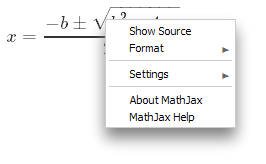#### Zoom feature

This feature allows users to see an enlarged image of the equation which may be useful to both partially sighted and everyone else if there is a lot of small detail.  This feature can be enabled centrally so that it is automatic for all users, or enabled via the options menu like this: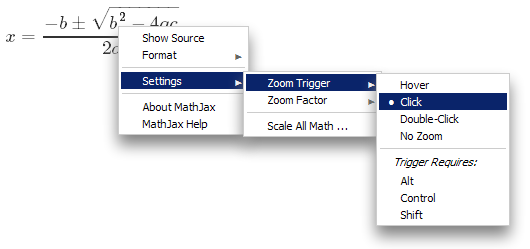With these settings, clicking on the equation now displays an enlarged version: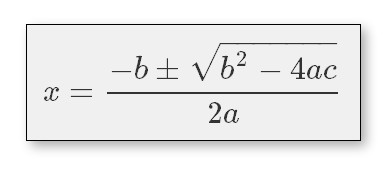#### Show Source

The Show Source feature unsurprisingly gives you access to a source version of the equation.  This is particularly useful as the source can be pasted into MathML compatible mathematical software such as Mathematica.  It can also be pasted into Microsoft Word (version 2007 and above) where the equation will be displayed as it should.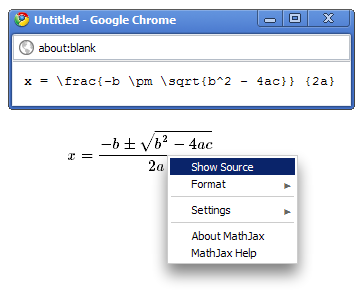Note that if the input notation is originally as LaTeX, by changing the Format option to “MathML”, the source can be viewed as MathML – MathJax translates the LaTeX into MathML.  However the reverse (MathML input to LaTeX is not possible).

### Where to go from here?

This article is just an introduction to using MathJax as a solution to displaying high-quality typeset mathematics within web pages.  However, you really you need to try it yourself to appreciate its strengths and limitations.  If you are interested in finding out more about MathJax or if you want to dive in and use it, but need help getting it set up, please get in touch at elearning@manchester.ac.uk.  We are happy to share the evaluation work we have done on MathJax to anyone interested.

Mathematical notation on the web – part 2
Tagged on:

### One thought on “Mathematical notation on the web – part 2”

•6th July 2015 at 6:41 am
Permalink

Thanks. Just what I was looking for. Well written.

Reply

This site uses Akismet to reduce spam. Learn how your comment data is processed.